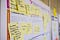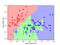# How To Improve Your Model’s Performance Using Cross-Validation TechniquesSource: Daria Nepriakhina On Unspalsh

Machine Learning models often fail to generalize well on data it has not been trained on. Hence, there is always a need to validate the stability of your machine learning model. It means we need to ensure that the efficiency of our model remains constant throughout. In other words, we need to validate how good our model is performing on unseen data. Based on the performance of model on unseen data, we can say whether model is overfitted, underfitted or well generalized.

## Why do models lose stability?

Let’s understand using below illustration:

# Machine Learning Fundamentals: Cosine Similarity and Cosine Distance

Cosine similarity is a metric that measures the cosine of the angle between two vectors projected in a multi-dimensional space.

The smaller the angle between the two vectors, the more similar they are to each other.

Suppose the angle between the two vectors is 90 degrees, the cosine similarity will have a value of 0; this means that the two vectors are perpendicular to each other which means they have no correlation between them.

As the cosine similarity measurement gets closer to 1, then the angle between the two vectors A and B becomes smaller. …

# Machine Learning Basics: K-Nearest Neighbours Python Implementation

The k-nearest neighbours (KNN) algorithm is a simple, easy-to-implement yet powerful supervised machine learning algorithm that can be used to solve classification problems and can be extended to regression problems.

## How KNN works under the hood?

K-nearest neighbours (KNN) algorithm uses ‘feature similarity’ to predict the values of new data points, which means that the new data point will be assigned a value based on how closely it matches the points in the training set.

The KNN algorithm assumes that similar things exist in close proximity. In other words, similar things are near to each other.Source: https://scipy-lectures.org/packages/scikit-learn/auto_examples/plot_iris_knn.html

Notice in the above plot how most of the similar…

# Fundamentals of Deep Learning — Activation Functions and When to Use Them?

Activation Functions are the most fundamental building blocks of deep learning. If you’re a beginner to deep learning like me, you must’ve had questions like “why do we have so many activation functions?”, “why does one works better than the other?”, “how do we know which one to use?”, “Should I be an expert in mathematics to understand them?” floating around in your mind. In this article, I’m going to answer all those whats, whys and hows. Let’s dive in.

I highly recommend that you understand what an artificial neural network is & how it works before you go through…

# Linear Regression From Scratch in Python WITHOUT Scikit-learn

In this tutorial, I’ll go over a brief introduction to one of the most commonly used machine learning algorithms, Linear Regression, and then we’ll learn how to implement it using the least-squares method from scratch in python without sci-kit-learn. We’ll also look at the interpretation of R squared in regression analysis and how it can be used to measure the goodness of the regression model.

Linear Regression is a type of predictive analysis algorithm that shows a linear relationship between the dependent variable(x) and independent variable(y).

Based on the given data points, we try to plot a straight line that…

# What Is Data Sampling and Statistical Techniques for Effective Sampling in Machine Learning

Data is the currency of the applied AI landscape. So it is of utmost importance that we make the best of data available and utilize it to draw practical and effective conclusions to solve real-world problems.

One of the biggest problems we face in applied machine learning is dealing with huge amounts of data on machines with limited computational power. Our machines are all too excited to throw the dreaded “out of memory” exception at us even when dealing with *slightly* large data sets.

So how do we overcome this persisting issue? Is there a way to select and analyze…## Sindhu Seelam

Transitioning ML/AI Engineer. I’m passionate about learning & writing about my journey into the AI world. https://www.linkedin.com/in/sindhuseelam/